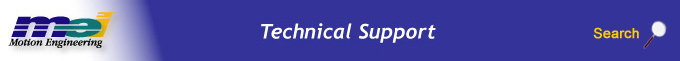.

MEIPostfilterSection

Definition

 ```typedef struct MEIPostfilterSection { MEIFilterType type; MEIFilterForm form; struct { struct { double breakPoint; /* Hz */ } lowPass; struct { double breakPoint; /* Hz */ } highPass; struct { double centerFrequency; /* Hz */ double bandwidth; /* Hz */ } notch; struct { double centerFrequency; /* Hz */ double bandwidth; /* Hz */ double gain; /* dB */ } resonator; struct { double lowFrequencyGain; /* dB */ double highFrequencyGain; /* dB */ double centerFrequency; /* Hz */ } leadLag; struct { double a1; double a2; double b0; double b1; double b2; } biquad; struct { double a1; double a2; double b0; double b1; double b2; } digitalBiquad; struct { long poleCount; long zeroCount; struct { double real; double imag; } pole; struct { double real; double imag; } zero; } polesZeros; struct { long poleCount; long zeroCount; struct { double real; double imag; } pole; struct { double real; double imag; } zero; } digitalPolesZeros; struct { double d1; double c1; double c2; double a2; double a1; double b1; } stateSpaceBiquad; } data; } MEIPostfilterSection;```

Description

MEIPostfilterSection holds the configuration data for a single section of an MPIFilter object's postfilter. The MPI calculates the post filter coefficients and takes into consideration the sample rate of the controller at that time. If you change the sample rate of the controller, you will need to recalculate the post filters. This can be done for all filters specified in Hertz by setting the filters again with the MPI. The MPI will calculate the filters using the current servo sample rate.

Postfilters are used to digitally filter the output of a control loop. One common use for postfilters is the compensation of system resonances.

 type The postfilter section type. This field determines which field of the MEIPostfilterSection.data union is used by meiFilterPostfilter.() methods. More information about particular filter types can be found below and in the MEIFilterType documentation. form The form of a postfilter section. The form determines how a particular postfilter section is calculated on the controller. All forms have certain limitations and tradeoffs. Please refer to MEIFilterForm for more information. lowPass.breakpoint The break point (measured in Hertz) of a low pass postfilter section.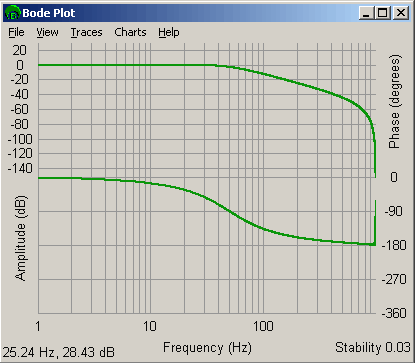Example of a 50 Hz low pass filter. highPass.breakpoint The break point (measured in Hertz) of a high pass postfilter section.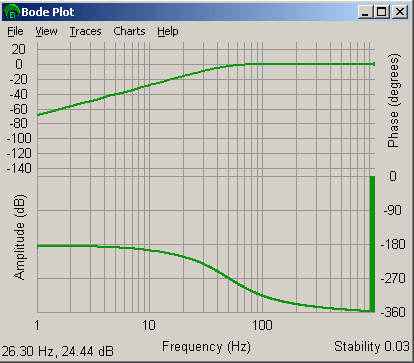Example of a 50 Hz High pass filter notch.centerFrequency The center frequency (measured in Hertz) of a notch postfilter section.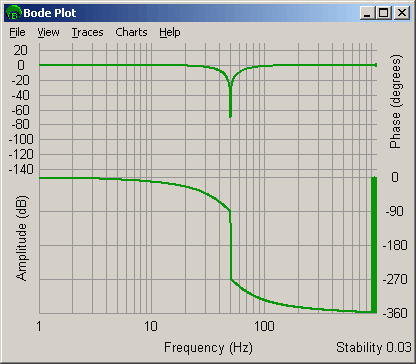Example of a 50 Hz Center / 50 Hz Bandwidth Notch filter. Note that phase wrapping gives the illusion that phase drops 180 degrees after the center frequency. The phase raises by 180 degrees. notch.bandwidth The bandwidth (measured in Hertz) of a notch postfilter section.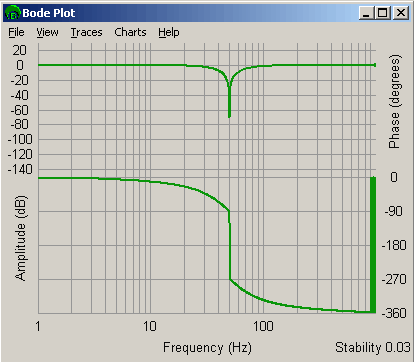Example of a 50 Hz Center / 50 Hz Bandwidth Notch filter. Note that phase wrapping gives the illusion that phase drops 180 degrees after the center frequency. The phase raises by 180 degrees. resonator.centerFrequency The center frequency (measured in Hertz) of a resonator postfilter section.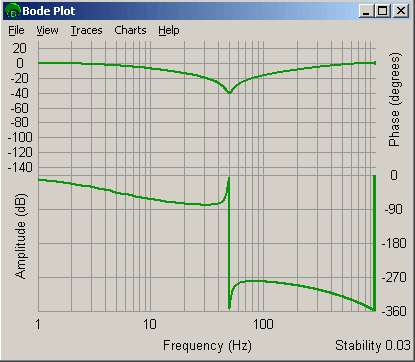Example of a 50 Hz center / 50 Hz Bandwidth / -40 dB Gain Resonator filter. Note that phase wrapping gives the illusion that the phase drops 360 degrees after the center frequency. resonator.bandwidth The bandwidth (measured in Hertz) of a resonator postfilter section.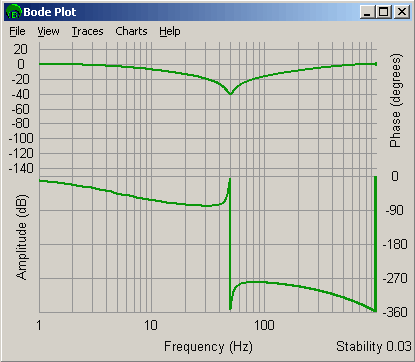Example of a 50 Hz center / 50 Hz Bandwidth / -40 dB Gain Resonator filter. Note that phase wrapping gives the illusion that the phase drops 360 degrees after the center frequency. resonator.gain The center frequency gain (measured in dB) of a resonator postfilter section.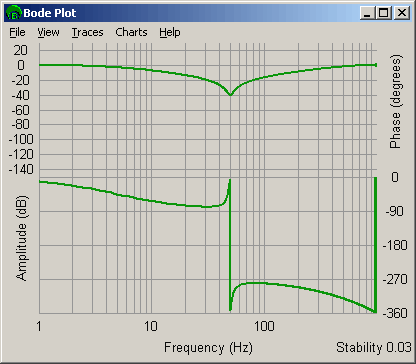Example of a 50 Hz center / 50 Hz Bandwidth / -40 dB Gain Resonator filter. Note that phase wrapping gives the illusion that the phase drops 360 degrees after the center frequency. leadLag.centerFrequency The center frequency (measured in Hertz) of a lead or lag postfilter section. The amplitude at this frequency is the average amplitude of the low and high frequency amplitudes. The gain (measured in dB) at this point is given by: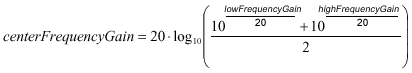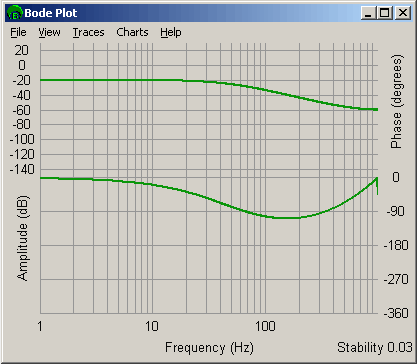Example of a -20 dB low frequency gain / -60 dB high frequency gain / 50 Hz center lead lag filter. leadLag.lowFrequencyGain The low frequency gain (measured in dB) of a lead or lag postfilter section.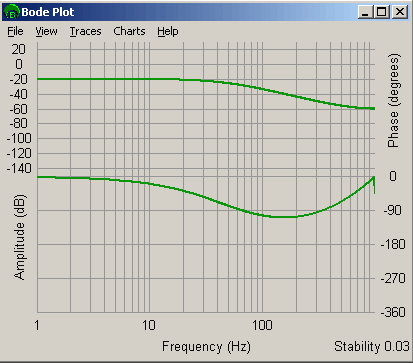Example of a -20 dB low frequency gain / -60 dB high frequency gain / 50 Hz center lead lag filter. leadLag.highFrequencyGain The high frequency gain (measured in dB) of a lead or lag postfilter section.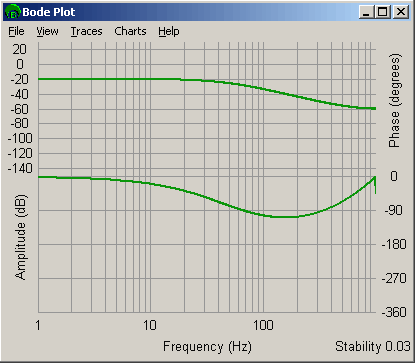Example of a -20 dB low frequency gain / -60 dB high frequency gain / 50 Hz center lead lag filter. biquad.a1 The analog coefficients of a single order or bi-quad postfilter section. Analog values of the postfilter coefficients are produced as parts of a Laplace Transform: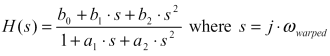and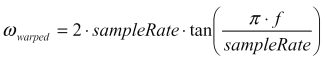biquad.a2 biquad.b0 biquad.b1 biquad.b2 digitalBiquad.a1 The digital coefficients of a single order or bi-quad postfilter section. digitalBiquad.a2 digitalBiquad.b0 digitalBiquad.b1 digitalBiquad.b2 digitalBiquad.d1 The digital coefficients of a state-space bi-quad postfilter section. digitalBiquad.c1 digitalBiquad.c2 digitalBiquad.a2 digitalBiquad.a1 digitalBiquad.b1 polesZeros.poleCount Analog poles and zeros. polesZeros.zeroCount polesZeros.pole[].real polesZeros.pole[].imag digitalPolesZeros.poleCount Digital poles and zeros. digitalPolesZeros.zeroCount digitalPolesZeros.pole[].real digitalpolesZeros.pole[].imag stateSpaceBiquad.d1 State space coefficients. stateSpaceBiquad.c1 stateSpaceBiquad.c2 stateSpaceBiquad.a2 stateSpaceBiquad.a1 stateSpaceBiquad.b1

Sample Code

 ```/* Set a 4th order low-pass post-filter by using two 2nd order low-pass sections. Sample usage: returnValue = fourthOrderLowPass(filter, 300 /* Hz */); */ long filterFouthOrderLowpass(MPIFilter filter, long breakPointFrequency) { MPIFilterConfig config; MEIPostfilterSection sections; long returnValue; section.type = MEIFilterTypeLOW_PASS; section.form = MEIFilterFormINT_BIQUAD; section.lowPass.breakpoint = breakPointFrequency; section = section; /* copy first section */ returnValue = meiFilterPostfilterSet(filter, 2, sections); return returnValue; }```